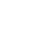Live chatAvon products foundation Inc. is a company incorporated in 1955. The mission of the company is to help improve the lives of women by bringing the mission through breast cancer and domestic violence as the main pillars. The foundation manages such programs like scholarship, emergency relief funds and associate matching gifts. This paper will calculate some of Avon’s financial ratios for the financials years 2008 and 2009.

The Year Ending December 2008

Gross Profit Margin

= (Sales – Cost of Goods Sold)/ Sales * 100%

= (\$10.59-\$3.94)/ \$10.59

= 0.63

Operating Profit Margin

= Operating Income/ Net Sales

= 13, 238/ 10,590

= 1.25

Net Profit Margin

= Net Profit/ Net Sales

= 875.3/ 10590

## Buy Free Custom «Avon Case Analysis» Essay Paper paper online

* Final order price might be slightly different depending on the current exchange rate of chosen payment system.

= 0.082

Times interest earned coverage

= (EBIT/ Annual Interest Expense) or (Net Income/ Annual Interest Expense)

= 875.3/ 100.4

= 8.72

Return on shareholder’s equity

= Net Income/ Average Shareholders Equity

= 875.3M/ 426.36

= 2.05

Return on assets

= Net Income/ Total Assets

= 875.3/ 26745.685

= 0.032

Debt-to-equity ratio

= (Long-Term Debt + Value of Leases)/ Average Shareholders Equity

= (1,456.2+ 3936.25)/ 674.9

= 7.99%

Days of inventory

= 365 days/ inventory turnover or (inventory/Cost of Goods Sold)* 365 days

= (335M/3.95B)*365 days

= 3.1

Inventory turnover ratio

= Sales/ Inventory or (COGS/Average Inventory)

= 10590/335

= 31.6

Average collection period

= Account Receivables/ (Annual Credit Sales/365 Days)

= 687.8/ (537.7/365days)

= 35

Stay ConnectedThe Year Ending December 2009

Gross profit margin

= (Sales – Cost of Goods Sold)/ Sales * 100%

= (10.28 – 3.89) * 100%

= 0.62

Operating profit margin

= Operating Income/ Net Sales

= 1280/625.8

= 2.05

Net profit margin

= Net Profit/ Net Sales

= 625.8/ 10,280

=0.06

Times interest earned coverage

= (Net Income/ Annual Interest Expense)

= 625.8/ 104.8

= 6.00

Return on shareholder’s equity

= Net Income/ Average Shareholders Equity

= 625.8M/ 426.9

= 1.47

Return on assets

= 625.8/ 30694.259

= 0.02

Debt-to-equity ratio

= (Long-Term Debt + Value of Leases)/ Average Shareholders Equity

= (1,256.8+2586.25)/426.9

= 9.2

Days of inventory

Limited time Offer

0
0
days
:
0
0
hours
:
0
0
minutes
:
0
0
seconds
Get 19% OFF

= 365 days/ inventory turnover or (inventory/Cost of Goods Sold)* 365 days

= (365/91.4)

= 4

Inventory turnover ratio

= Sales/ Inventory or (COGS/Average Inventory)

= 10.28B/298.3M

= 34.46

Average collection period

= Account Receivables/ (Annual Credit Sales/365 Days)

= 779.7/ (456.5/365)

=64.9

Based on these ratios, did Avon’s financial performance improve, weaken or remain about the same from 2008 to 2009?

From the above ratios, we can conclude that Avon’s financial performance weakened slightly in 2009 than it was in 2008 as shown by a fall in financial ratios. This may be attributed to the 2008 financial crisis whose toll was well felt in 2009.

#### Related Analysis essays

0

Preparing Orders

0

Active Writers

0

Support Agents

Limited offerget 15% off your 1st order